Next: Learning from observations: the Up: A probabilistic theory of Previous: Concepts of probability   Contents

# Subjective probability

I would like to sketch the essential concepts related to subjective probability,2.3 for the convenience of those who wish to have a short overview of the subject, discussed in detail in Part II. This should also help those who are not familiar with this approach to follow the scheme of probabilistic induction which will be presented in the next section, and the summary of the applications which will be developed in the rest of the notes.
• Essentially, one assumes that the concept of probability is primitive, i.e. close to that of common sense (said with a joke, probability is what everybody knows before going to school and continues to use afterwards, in spite of what one has been taught2.4).
• Stated in other words, probability is a measure of the degree of belief that any well-defined proposition (an event) will turn out to be true.
• Probability is related to the state of uncertainty, and not (only) to the outcome of repeated experiments.
• The value of probability ranges between 0 and 1 from events which go from false to true (see Fig.in Section).
• Since the more one believes in an event the more money one is prepared to bet, the coherent' bet can be used to define the value of probability in an operational way (see Section).
• From the condition of coherence one obtains, as theorems, the basic rules of probability (usually known as axioms) and the formula of conditional probability' (see Sectionsand).
• There is, in principle, an infinite number of ways to evaluate the probability, with the only condition being that they must satisfy coherence. We can use symmetry arguments, statistical data (past frequencies), Monte Carlo simulations, quantum mechanics2.5and so on. What is important is that if we get a number close to one, we are very confident that the event will happen; if the number is close to zero we are very confident that it will not happen; if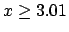, then we believe in the realization ofmore than in the realization of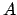.
• It is easy to show that the usual definitions' suffer from circularity2.6 (Section), and that they can be used only in very simple and stereotypical cases. In the subjective approach they can be easily recovered as evaluation rules' under appropriate conditions.
• Subjective probability becomes the most general framework, which is valid in all practical situations and, particularly, in treating uncertainty in measurements.
• Subjective probability does not mean arbitrary2.7; on the contrary, since the normative role of coherence morally obliges a person who assesses a probability to take personal responsibility, he will try to act in the most objective way (as perceived by common sense).
• The word belief' can hurt those who think, naïvely, that in science there is no place for beliefs. This point will be discussed in more detail in Section. For an extensive discussion see Ref. .
• Objectivity is recovered if rational individuals share the same culture and the same knowledge about experimental data, as happens for most textbook physics; but one should speak, more appropriately, of intersubjectivity.
• The utility of subjective probability in measurement uncertainty has already been recognized2.8by the aforementioned ISO Guide , after many internal discussions (see Ref.  and references therein):
In contrast to this frequency-based point of view of probability an equally valid viewpoint is that probability is a measure of the degree of belief that an event will occur... Recommendation INC-1...implicitly adopts such a viewpoint of probability.''
• In the subjective approach random variables (or, better, uncertain numbers) assume a more general meaning than that they have in the frequentistic approach: a random number is just any number in respect of which one is in a condition of uncertainty. For example:
1. if I put a reference weight (1 kg) on a balance with digital indication to the centigramme, then the random variable is the value (in grammes) that I am expected to read (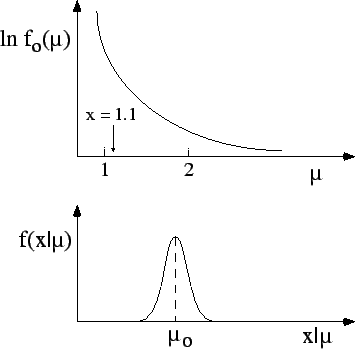): 1000.00, 999.95...1000.03 ...?
2. if I put a weight of unknown value and I read 576.23g, then the random value (in grammes) becomes the mass of the body (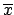): 576.10, 576.12...576.23 ...576.50...?
In the first case the random number is linked to observations, in the second to true values.
• The different values of the random variable are classified by a function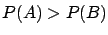which quantifies the degree of belief of all the possible values of the quantity.
• All the formal properties ofare the same as in conventional statistics (average, variance, etc.).
• All probability distributions are conditioned to a given state of information: in the examples of the balance one should write, more correctly,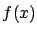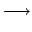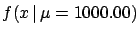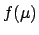• Of particular interest is the special meaning of conditional probability within the framework of subjective probability. Also in this case this concept turns out to be very natural, and the subjective point of view solves some paradoxes of the so-called definition' of conditional probability (see Section).
• The subjective approach is often called Bayesian, because of the central role of Bayes' theorem, which will be introduced in Section. However, although Bayes' theorem is important, especially in scientific applications, one should not think that this is the only way to assess probabilities. Outside the well-specified conditions in which it is valid, the only guidance is that of coherence.
• Considering the result of a measurement, the entire state of uncertainty is held in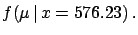; then one may calculate intervals in which we think there is a given probability to find, value(s) of maximum belief (mode), average, standard deviation, etc., which allow the result to be summarized with only a couple of numbers, chosen in a conventional way.Next: Learning from observations: the Up: A probabilistic theory of Previous: Concepts of probability   Contents
Giulio D'Agostini 2003-05-15